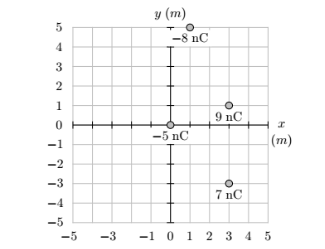# Problem: Four charges −5×10−9 C at (0m, 0m), −8×10−9 C at (1m, 5m), 9×10−9 C at (3m, 1m), and 7×10−9 C at (3m, −3m), are arranged in the ( x, y ) plane (as shown in the figure below, where the scale is in meters).Find the magnitude of the resulting force on the −5 nC charge at the origin [coordinates, (0m, 0m)].

###### FREE Expert Solution
90% (386 ratings)
###### Problem Details

Four charges −5×10−9 C at (0m, 0m), −8×10−9 C at (1m, 5m), 9×10−9 C at (3m, 1m), and 7×10−9 C at (3m, −3m), are arranged in the ( x, y ) plane (as shown in the figure below, where the scale is in meters).

Find the magnitude of the resulting force on the −5 nC charge at the origin [coordinates, (0m, 0m)].